### IMO Shortlist 1978 problem 1

Kvaliteta:
Avg: 0,0
Težina:
Avg: 0,0
The set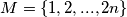$M = \{1, 2, . . . , 2n\}$ is partitioned into$k$ nonintersecting subsets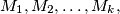$M_1,M_2, \dots, M_k,$ where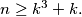$n \ge k^3 + k.$ Prove that there exist even numbers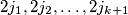$2j_1, 2j_2, \dots, 2j_{k+1}$ in$M$ that are in one and the same subset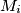$M_i$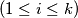$(1 \le i \le k)$ such that the numbers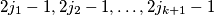$2j_1 - 1, 2j_2 - 1, \dots, 2j_{k+1} - 1$ are also in one and the same subset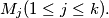$M_j (1 \le j \le k).$
Izvor: Međunarodna matematička olimpijada, shortlist 1978